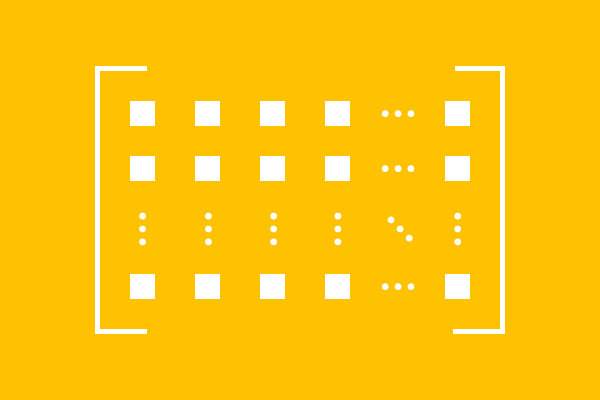# Rectangular Matrix

A matrix whose number of rows does not equal to the number of columns, is called a rectangular matrix.

## IntroductionRectangular matrix is a type of matrix and the elements are arranged in the matrix as number of rows and the number of columns. The arrangement of elements in matrix is in rectangle shape. Thus, it is called as a rectangular matrix.

The rectangular matrix can be expressed in general form as follows. The elements of this matrix are arranged in $m$ rows and $n$ columns. Therefore, the order of the matrix is $m \times n$.

$$M = {\begin{bmatrix} e_{11} & e_{12} & e_{13} & \cdots & e_{1n}\\ e_{21} & e_{22} & e_{23} & \cdots & e_{2n}\\ \vdots & \vdots & \vdots & \ddots & \vdots \\ e_{m1} & e_{m2} & e_{m3} & \cdots & e_{mn} \end{bmatrix}}_m \times n}$$

Rectangle shape in matrix is possible if the number of rows is different to the number of columns. It means $m \ne n$. Therefore, there are two possibilities to form rectangular matrix, one is number of rows is greater than the number of columns ($m > n$) and the other is number of rows is less than the number of columns ($m < n$).

### Example

The following two cases are the possibility for the formation of rectangular matrices in the matrix algebra.

01

#### Rows less than Columns

$A$ is a matrix and elements are arranged in matrix as $3$ rows and $4$ columns.

$$A = \begin{bmatrix} 5 & -1 & 4 & 9\\ -7 & 1 & 3 & 2\\ 8 & 5 & 0 & -6 \end{bmatrix}$$

The order of the matrix $A$ is $3 \times 4$. The number of rows is not equal to the number of columns ($3 \ne 4$), and also the number of rows is less than number of columns ($3 < 4$). Therefore, the matrix $A$ is an example for a rectangular matrix.

02

#### Rows greater than Columns

$B$ is a matrix and the elements are arranged in the matrix in $5$ rows and $2$ columns.

$$B = \begin{bmatrix} 2 & 6\\ 5 & 2\\ 9 & 4\\ 6 & 2\\ 7 & -6 \end{bmatrix}$$

The order of the matrix $B$ is $5 \times 2$. The number of rows is not equal to the number of columns ($5 \ne 2$) and also the number of rows is greater than number the columns ($5 > 2$). So, the matrix $B$ is known as a rectangular matrix.

Latest Math Topics
Email subscription
Math Doubts is a best place to learn mathematics and from basics to advanced scientific level for students, teachers and researchers. Know more